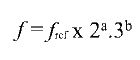One-dimensional Tone Factory

The One-dimensional Tone Factory works to the specification:where fref is the reference frequency, and a and b are positive or negative integers. The value of b is sufficient in this instance to determine the position of a tone along the single dimension. The value of a has been fixed in order to give f a value between 1 and 2. Disabling the octave dimension (powers of 2) by this means allows us to map this space to one rather than two dimensions.

close